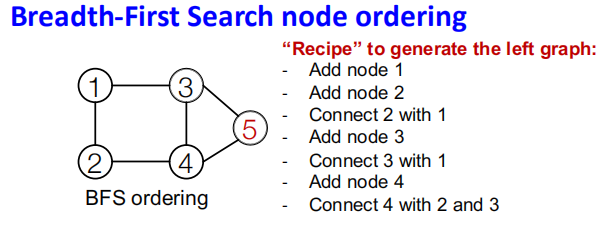# Stanford图机器学习公开课CS224W（十）笔记

## Lecture 10 -Deep Generative Models for Graphs

Posted by Yunlongs on December 8, 2020

# Deep Generative Models for Graphs

## 一.图生成模型的难点所在

1. 非常大的变量输出空间

1. 图不唯一的表征

n个节点的图通过节点顺序的置换，可以表征成$n!$个不同的邻接矩阵

1. 节点间复杂的依赖关系

## 二.Recap:Generative Models

Given: 从$p_{data}(G)$中采样的图样本

Goal： 从数据中学习出分布$p_{model}(G)$，然后从中采样。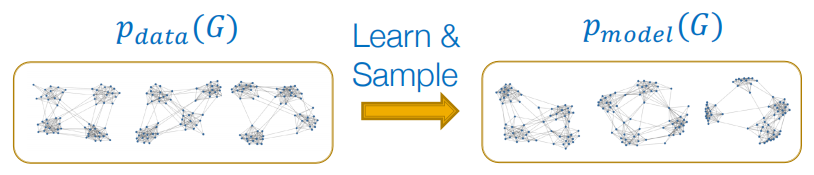(1)首先要使$p_{model}(x;\theta)$与真实的数据分布$p_{data}(x)$分布足够接近

$\boldsymbol{\theta}^{*}=\underset{\boldsymbol{\theta}}{\arg \max } \mathbb{E}_{x \sim p_{\text {data }}} \log p_{\text {model }}(\boldsymbol{x} \mid \boldsymbol{\theta})$

(2)从训练好的$p_{model}(x;\theta)$中进行采样

$p_{\text {model}}(\boldsymbol{x} ; \theta)=\prod_{t=1}^{n} p_{\text {model}}\left(x_{t} \mid x_{1}, \ldots, x_{t-1} ; \theta\right)$

## 三.GraphRNN: Generating Realistic Graphs

### 3.1 Model Graphs as Sequences

GraphRNN的主要思想就是，把图生成的过程当成为添加节点、添加边的序列生成过程。

1. 节点级别： 每个时间步添加一个节点
2. 边级别： 每添加一个节点时，就添加此节点与已存在节点之间的边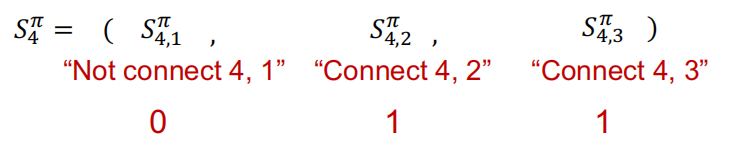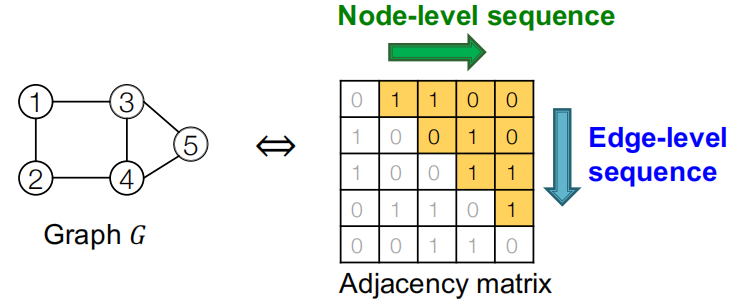### 3.2 GraphRNN: Two Levels of RNN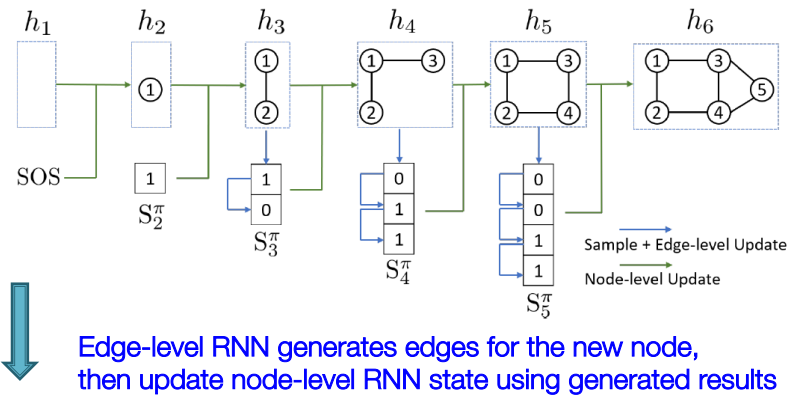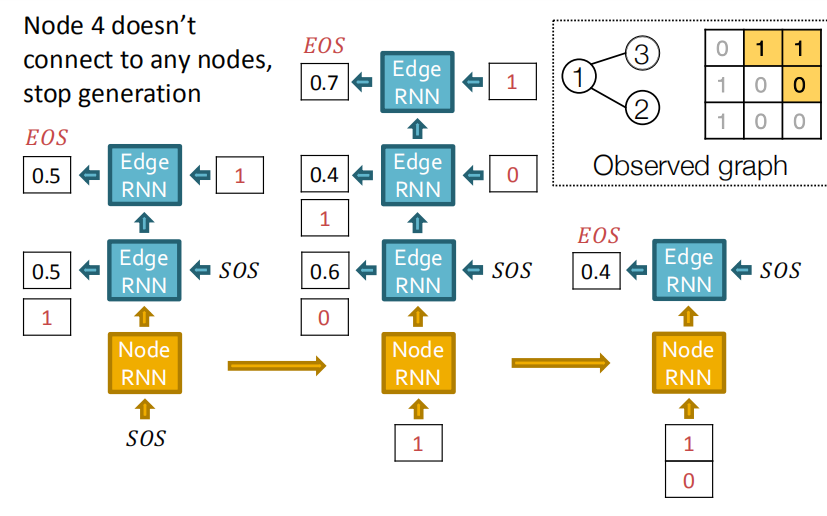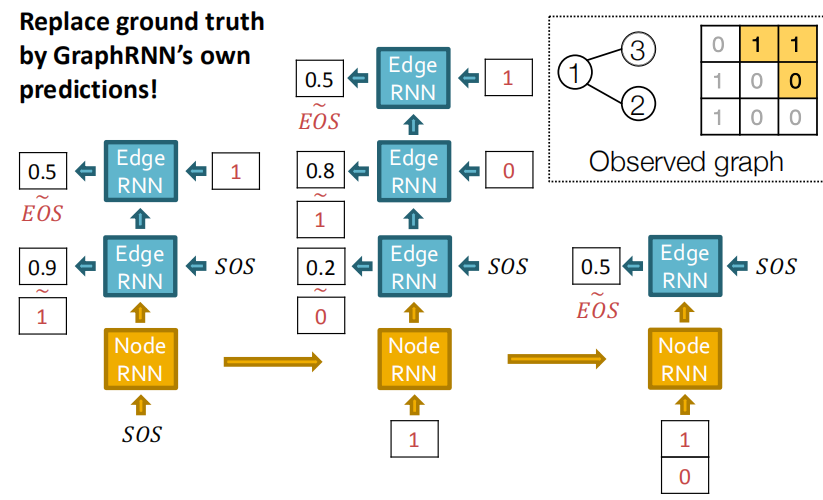### 3.3 Issue：Tractability

BFS的节点序带来的好处也是显而易见的，降低了边生成所需要的步数，每个节点只需要考虑局部的邻接信息就行。